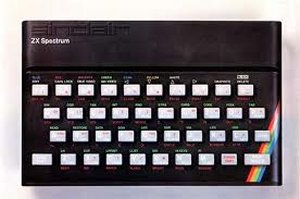BINARY

I should precede this section with the words "Don't Panic". Binary is actually very easy and, in any case, the computer uses it not you. My car eats petrol but that does not mean I have to try it as well!

We use the decimal system, meaning 10. In fact, we actually use the numbers zero to nine. After 9, things change substantially. We have run out of our numbers so we actually say that we now have one lot of 0-9 and start again at zero. So, I start with zero old computers, then one, two, three and so on until I have nine. Now I collect one more so I have one lot of my ten numbers plus zero more or 10 (ten). Another nine later (19) then one and I have two lots of 10 so 20. We all do this every day and never give it a moment's thought.

Binary is exactly the same but everything happens much quicker. I start with zero computers. I buy one so I now have 1 computer. Now I buy another and ----

I can only add up to one so now I have zero plus one lot of 0-1 therefore 10 (read it as one, nought). I buy another and I have 11 (one lot of 2 plus one more) then another makes 100 (one, nought, nought) in the same way that 100 follows 99.

As I said, don't panic, you do not need to be able to work in binary but it helps me explain the significance of the magic 8 bits. 8 bits means exactly that, numbers are worked on as 8 bits so (technically) 00000000 (zero) to 11111111 (255), for the clever amongst you, 2 to the power of 8 minus 1. If that is insufficient then you can work on more than one lot of 8 bits; 2 sets of 8 gives 65536 and so on.

So, armed with our new-found understanding of bit and binary, what is an 8 bit computer? .